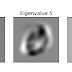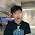## March 9, 2016 (Wed) - March 10, 2016 (Thu)

Finished my school's first programming assignment.

I decided to ditch my machine learning for some time since I also had my FYP and networking class to handle (and I was still trying to squeeze to continue my nanodegree study...), but I had to go back in early March to meet the deadline of my school's first coding assignment. I hadn't been to lecture for ages so I still panicked a little bit when it was released. But after skimming the specification, I was really relieved it was such a simple assignment. Malone kinda already went though the basics I needed to know in her coding and mini-project videos.

As a start, I needed to explore the Boston dataset in Scikit-learn to do something about Linear Regression. Then I moved on to explore some raw data (which meant I had to do some data preprocessing myself) and did pretty much the same thing. But this time, apart from only harnessing the mighty power of Scikit-learn, I also had to wrote my own code to implement linear regression, which was only a bunch of mathematical operations, nothing Python and I couldn't handle.

Let's say our linear regression model is y = X'(theta). So our goal is to find theta, i.e. the coefficients. With this equation,

See? A bunch of dot(), inv() and tranpose() in Python are all you need.

Since we were already on linear regression, why didn't we add Ridge Regression into the mix to have more fun? I bet it was what the prof said when he designed this assignment. The problem was, I didn't know Ridge Regression back then. Luckily, Scikit-learn was designed for people like us, people who didn't know machine learning but tried to do machine learning, and then told people they knew machine learning. Therefore, I successfully made it to the final stage: binary classification using logistic regression, which was also something I didn't know, but Scikit-learn saved my life once again. So yay!

You can check out my code and spec here: https://github.com/kevguy/CSCI3320Asg1

Note: Laugh all you want, but I finally learned Ridge Regression and Logistic Regression a couple weeks later, when I had the time.

## Mar 25, 2016 (Fri) - Mar 27, 2016 (Sun)

Finished my school's second programming assignment. Finished Lesson 12 (sort of).

When I said I ditched my machine learning study, I really meant it. Let's see what I did this couple weeks. Mm... I coded a proxy for my networking class, spent some time studying some crap for a crappy class called "Technology, Society and Engineering Practice", got a midterm today (even the prof admitted he taught this class only because it was a requirement from our faulty). And I did some work on my FYP using NetworkX, what an awesome library!

Beyond doubt I was even more terrified when I knew the second coding assignment was released. PCA? Never heard of it. Oh...Principal component analysis huh? What does it do? I quickly went back to Udacity to see if Thurn and Malone did a lesson about it... and SCORE! So I managed to learn the basics of what PCA does in half an hour. I don't have time to finish the mini-project yet, but still, Udacity I love you so much! After skimming the lecture notes I had from my school and panicked after seeing all those maths, I thought I was ready to get my hands dirty.

My first task was to perform PCA using a generated dataset (a 1000x9 matrix), the catch was I had to write my own PCA. Here's what I did (let's say the matrix I got is called X).

1.  Calculate the mean vector (i.e. get the mean for every freakin' column of X)

sum_X  = [[ sum(x) for x in zip(*X) ]]
sum_X = np.asarray(sum_X)

# Calculate the mean vector
mean_vector = sum_X / 1000.0



2. Normalize the crap outta matrix X using the mean_vector I got

# Normalize the whole thing
thousand_ones = np.ones((1000, 1))
X_normalized = np.dot(thousand_ones, mean_vector)
X_normalized = np.subtract(X, X_normalized)


3. Now we're all set to calculate the covariance matrix,

S = np.cov(np.transpose(X_normalized))


4. And finally, get our eigenvalues and eigenvectors

[eig_vals, eig_vecs] = np.linalg.eig(S)

The second task was a little bit more fun, although I really didn't see the point of doing it. I got a dataset full of images of digits 0, 1, and 2, and my job was to apply PCA and plot the eigenfaces (I think eigendigits would be a more appropriate term, whatever) and finally calculate the proportion of variance. And here are my results:

I thought I would do something more fun, like testing and validation, like face recognition (or digit recognition here). Also, I Googled for a whole day and I don't think it has any meaning finding the POV here, because I almost don't see any people doing that. Anyway, you can check out my code and spec here: https://github.com/kevguy/CSCI3320Asg2

Finally, here are some useful links about PCA if you wanna know more:

## Mar 29, 2016 (Tue)

Finished Lesson 8 and 9.

Today is the quiz for the crappy course. After studying some more in the morning, I gave up. I just couldn't take it any more, so I switched back to finish mini-project for Lesson 8. I guess it was because I was really tired, so I couldn't quite understand what Thurn and Malone were talking about when they were explaining what k-means was doing. I finally figured it out when I was doing the stupid quiz, yeah you heard me right. The quiz, which would contribute to one-fourth of my grade, consisted of 10 multiple choice questions and two five-point short questions. I finished the whole thing in ten minutes because I really didn't know what was going on. Nevermind, I got the next 50 minutes for myself. After some doodling around, I couldn't believe it took me that long to figure out such a simple algorithm:

1. Input: K, which consists a set of points x1, ...xn
2. Place centroids c1, c2, ..., ck at random locations
3. Repeat until convergence:
1. for each point xi:
1. find nearest centroid cj (i.e. argmin D(xi, cj)) and assign the point xi to cluster cj
2. for each cluster j = 1, 2, ...k
1. new centroid cj = mean of all points xi assigned to cluster j in previous step

And just so you know, I spent the rest of the time finishing a sudoku puzzle I memorized before going to the lecture, and then napped.

After going home, I finished the code for Lesson 8 (Clustering), you can check out the code here:
https://github.com/kevguy/Intro-to-Machine-Learning-ud120/tree/Stage08_04_Salary_Range

And Lesson 9 (Feature Scaling) was even easier, check out the code here:
https://github.com/kevguy/Intro-to-Machine-Learning-ud120/tree/Stage09_01_Computing_Rescaled_Features

I need to take some rest right now, and I guess I will go back to the books a little bit tomorrow.

Kev
My Machine Learning Learning Experience (Part 2): Assignments and UD120 Lesson 8 - 9Reviewed by Unknown on 8:00:00 AM Rating: 5

#### 1 comment:

1.